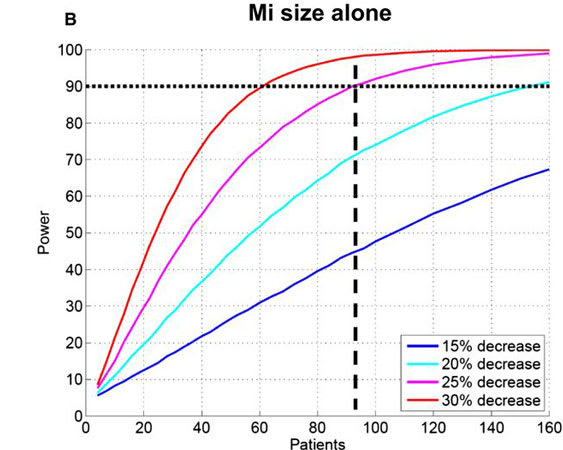Statistical power, within the context of hypothesis testing, is the probability of rejecting the test hypothesis at a specified level, given that the alternative hypothesis is true. In simpler words, it’s the probability of finding a statistically significant effect, when there is one.

Misconception: Statistical power can only be increased with larger sample sizes

Truth: Nope. Here are some other things that increase statistical power:

• Using specific variables (continuous or ordinal variables rather than categorical ones)

• Running analyses such as ANCOVA to reduce within-group variance

• Using within-subjects designs

• Looking for larger effects

• Setting larger alpha levels

• Reducing random error of your measurements

Misconception: You’ve reached enough statistical power, or your study is underpowered.

Truth: Statistical power is not some cutoff, it’s more like a curve. See below.Misconception: The problem with low power is that you’ll miss true effects.

Truth: While this is true with regards to statistical significance, studies that are underpowered will also produce statistically significant effects that are less likely to be true, (keep in mind that the false-positive rate is fixed), in that the point estimates are more influenced by noise and variability, thus, only the largest effects will pass your thresholds. Once those thresholds have been crossed, you will be fooled into thinking you’ve found a real significant effect.

Misconception: Effect sizes and standard deviations from pilot studies should be used to calculate sample sizes for larger studies.

Truth: Pilot studies are mainly done to see how studies will play out and to figure out what types of problems you will encounter with larger studies. Due to the small samples, pilot studies yield noisy point estimates and standard deviations that are likely to be far from the true population effects (probably an overestimate, see above, thus resulting in underpowered studies).

That’s all for today folks.